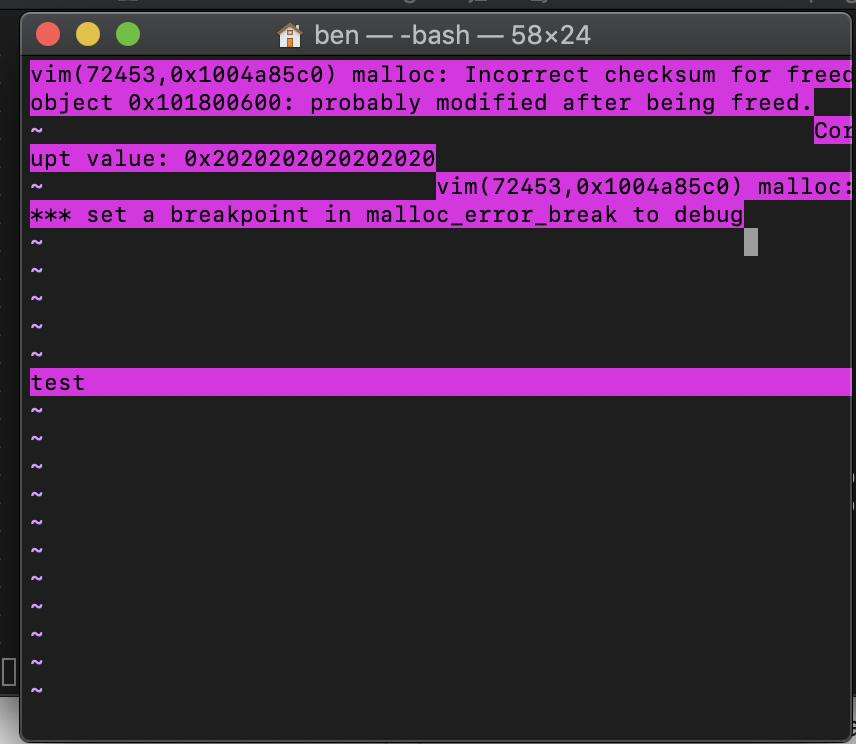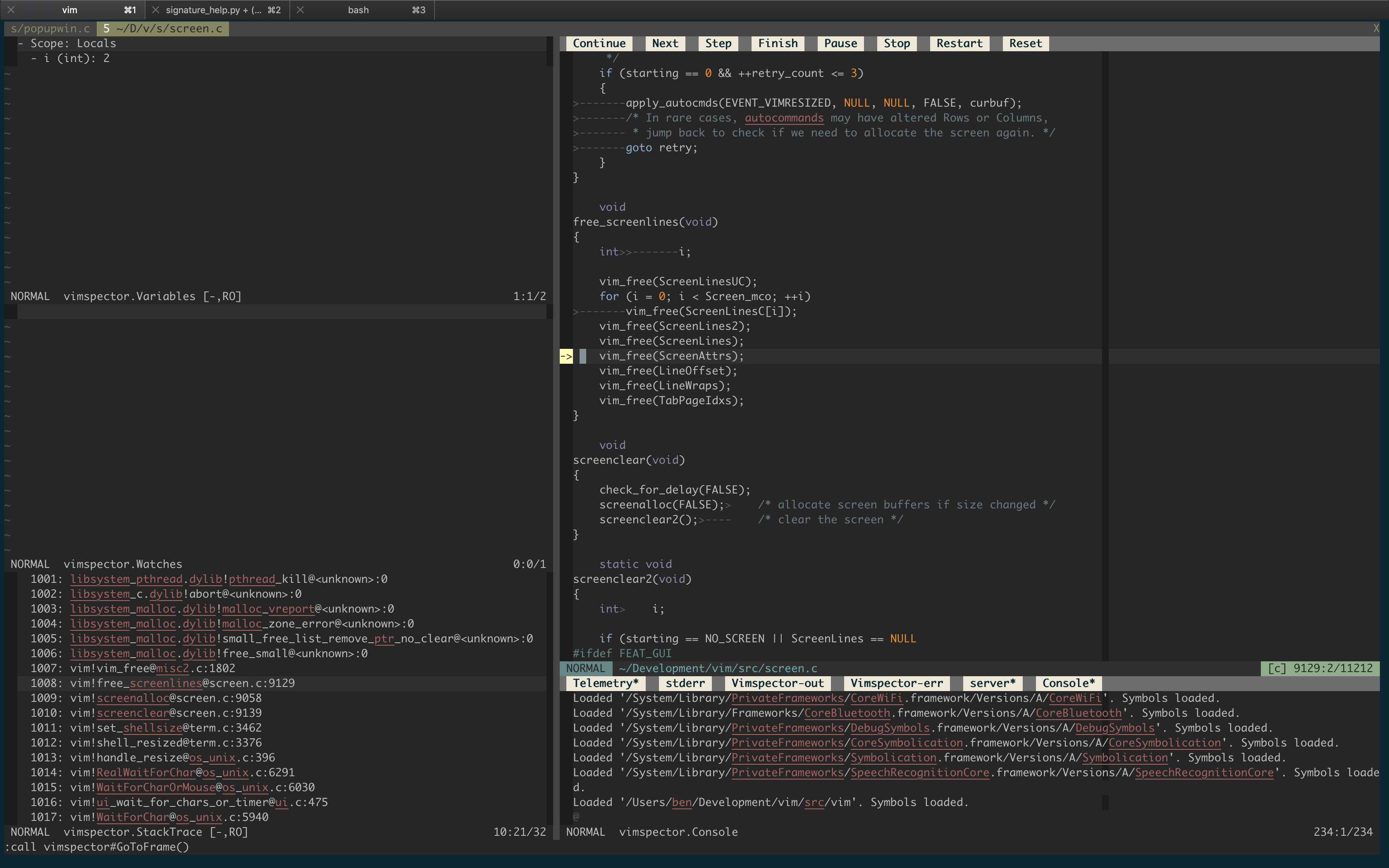Crash when resizing terminal with popup visible #4467

Closed
opened this issue Jun 1, 2019 · 8 comments

Projects
None yet

puremourning commented Jun 1, 2019

 Describe the bug Vim crashes when resizing the terminal with a popup visible. To Reproduce Detailed steps to reproduce the behavior: `vim -Nu NONE --clean --noplugin` `:call popup_create( 'test', { 'minwidth': 100 } )` resize the windows to a very small width Crash seems to be reallocating the screen buffers: Expected behavior No crash Screenshots Seems to happen when re-allocating the screen. May not be popup related but I found it while testing popups.Stack trace: ``````- Thread: Thread #1 1000: libsystem_kernel.dylib!__pthread_kill@:0 1001: libsystem_pthread.dylib!pthread_kill@:0 1002: libsystem_c.dylib!abort@:0 1003: libsystem_malloc.dylib!malloc_vreport@:0 1004: libsystem_malloc.dylib!malloc_zone_error@:0 1005: libsystem_malloc.dylib!small_free_list_remove_ptr_no_clear@:0 1006: libsystem_malloc.dylib!free_small@:0 1007: vim!vim_free@misc2.c:1802 1008: vim!free_screenlines@screen.c:9129 1009: vim!screenalloc@screen.c:9058 1010: vim!screenclear@screen.c:9139 1011: vim!set_shellsize@term.c:3462 1012: vim!shell_resized@term.c:3376 1013: vim!handle_resize@os_unix.c:396 1014: vim!RealWaitForChar@os_unix.c:6291 1015: vim!WaitForCharOrMouse@os_unix.c:6030 1016: vim!ui_wait_for_chars_or_timer@ui.c:475 1017: vim!WaitForChar@os_unix.c:5940 1018: vim!inchar_loop@ui.c:383 1019: vim!mch_inchar@os_unix.c:388 1020: vim!ui_inchar@ui.c:231 1021: vim!inchar@getchar.c:3088 1022: vim!vgetorpeek@getchar.c:2866 1023: vim!vgetc@getchar.c:1602 1024: vim!safe_vgetc@getchar.c:1817 1025: vim!normal_cmd@normal.c:596 1026: vim!main_loop@main.c:1363 1027: vim!vim_main2@main.c:903 1028: vim!main@main.c:444 1029: libdyld.dylib!start@:0 1030: libdyld.dylib!start@:0 `````` I got asan output too, but it's hard to capture due to resizing of the terimal windows. Environment (please complete the following information): Vim version 8.1.1436 OS: macOS 10.14 Terminal: Terminal.app

dpelle commented Jun 1, 2019

 @puremourning wrote: I got asan output too, but it's hard to capture due to resizing of the terimal windows. I can reproduce it with asan or valgrind. Just redirect stderr to capture asan or valgrind output. I used: ``````\$ valgrind ./vim --clean \ -c "call popup_create('test', {'minwidth': 100})" \ -c 'set columns=40' 2> valgrind.log `````` And valgrind.log contains: ``````==9362== Memcheck, a memory error detector ==9362== Copyright (C) 2002-2017, and GNU GPL'd, by Julian Seward et al. ==9362== Using Valgrind-3.13.0 and LibVEX; rerun with -h for copyright info ==9362== Command: ./vim --clean -c call\ popup_create('test',\ {'minwidth':\ 100}) -c set\ columns=40 ==9362== ==9362== Invalid write of size 1 ==9362== at 0x2909CD: win_line (screen.c:5824) ==9362== by 0x296DB2: win_update (screen.c:2156) ==9362== by 0x299029: update_popups (screen.c:1039) ==9362== by 0x299029: update_screen (screen.c:827) ==9362== by 0x34F8AB: main_loop (main.c:1256) ==9362== by 0x35095E: vim_main2 (main.c:903) ==9362== by 0x14564E: main (main.c:444) ==9362== Address 0x11d86d80 is 0 bytes after a block of size 2,800 alloc'd ==9362== at 0x4C2FB0F: malloc (in /usr/lib/valgrind/vgpreload_memcheck-amd64-linux.so) ==9362== by 0x212F80: lalloc (misc2.c:924) ==9362== by 0x287E4B: screenalloc (screen.c:8925) ==9362== by 0x288811: screenclear (screen.c:9139) ==9362== by 0x2DA9E4: set_shellsize (term.c:3462) ==9362== by 0x243B33: set_num_option (option.c:9426) ==9362== by 0x24D7F7: do_set (option.c:4828) ==9362== by 0x1B45C0: do_one_cmd (ex_docmd.c:2499) ==9362== by 0x1B45C0: do_cmdline (ex_docmd.c:994) ==9362== by 0x350B0F: exe_commands (main.c:2968) ==9362== by 0x350B0F: vim_main2 (main.c:797) ==9362== by 0x14564E: main (main.c:444) ==9362== ==9362== Invalid write of size 4 ==9362== at 0x290A0D: win_line (screen.c:5848) ==9362== by 0x296DB2: win_update (screen.c:2156) ==9362== by 0x299029: update_popups (screen.c:1039) ==9362== by 0x299029: update_screen (screen.c:827) ==9362== by 0x34F8AB: main_loop (main.c:1256) ==9362== by 0x35095E: vim_main2 (main.c:903) ==9362== by 0x14564E: main (main.c:444) ==9362== Address 0x11d89980 is 0 bytes after a block of size 11,200 alloc'd ==9362== at 0x4C2FB0F: malloc (in /usr/lib/valgrind/vgpreload_memcheck-amd64-linux.so) ==9362== by 0x212F80: lalloc (misc2.c:924) ==9362== by 0x28836B: screenalloc (screen.c:8929) ==9362== by 0x288811: screenclear (screen.c:9139) ==9362== by 0x2DA9E4: set_shellsize (term.c:3462) ==9362== by 0x243B33: set_num_option (option.c:9426) ==9362== by 0x24D7F7: do_set (option.c:4828) ==9362== by 0x1B45C0: do_one_cmd (ex_docmd.c:2499) ==9362== by 0x1B45C0: do_cmdline (ex_docmd.c:994) ==9362== by 0x350B0F: exe_commands (main.c:2968) ==9362== by 0x350B0F: vim_main2 (main.c:797) ==9362== by 0x14564E: main (main.c:444) ==9362== ==9362== Invalid write of size 2 ==9362== at 0x290A28: win_line (screen.c:5852) ==9362== by 0x296DB2: win_update (screen.c:2156) ==9362== by 0x299029: update_popups (screen.c:1039) ==9362== by 0x299029: update_screen (screen.c:827) ==9362== by 0x34F8AB: main_loop (main.c:1256) ==9362== by 0x35095E: vim_main2 (main.c:903) ==9362== by 0x14564E: main (main.c:444) ==9362== Address 0x11d907a0 is 0 bytes after a block of size 5,600 alloc'd ==9362== at 0x4C2FB0F: malloc (in /usr/lib/valgrind/vgpreload_memcheck-amd64-linux.so) ==9362== by 0x212F80: lalloc (misc2.c:924) ==9362== by 0x287E9E: screenalloc (screen.c:8936) ==9362== by 0x288811: screenclear (screen.c:9139) ==9362== by 0x2DA9E4: set_shellsize (term.c:3462) ==9362== by 0x243B33: set_num_option (option.c:9426) ==9362== by 0x24D7F7: do_set (option.c:4828) ==9362== by 0x1B45C0: do_one_cmd (ex_docmd.c:2499) ==9362== by 0x1B45C0: do_cmdline (ex_docmd.c:994) ==9362== by 0x350B0F: exe_commands (main.c:2968) ==9362== by 0x350B0F: vim_main2 (main.c:797) ==9362== by 0x14564E: main (main.c:444) ==9362== ==9362== Invalid read of size 1 ==9362== at 0x28B6A8: screen_line (screen.c:6524) ==9362== by 0x2946C6: win_line (screen.c:5728) ==9362== by 0x296DB2: win_update (screen.c:2156) ==9362== by 0x298E54: update_screen (screen.c:787) ==9362== by 0x2996CE: redraw_asap (screen.c:381) ==9362== by 0x2DF523: check_termcode (term.c:5016) ==9362== by 0x1DEC89: vgetorpeek.part.10 (getchar.c:2341) ==9362== by 0x1DFD42: vgetorpeek (getchar.c:2001) ==9362== by 0x1DFD42: vgetc (getchar.c:1602) ==9362== by 0x1E0168: safe_vgetc (getchar.c:1817) ==9362== by 0x22EFCD: normal_cmd (normal.c:596) ==9362== by 0x34F676: main_loop (main.c:1363) ==9362== by 0x35095E: vim_main2 (main.c:903) ==9362== Address 0x12e6639e is not stack'd, malloc'd or (recently) free'd ==9362== ==9362== ==9362== Process terminating with default action of signal 11 (SIGSEGV) ==9362== at 0x8DAF187: kill (syscall-template.S:78) ==9362== by 0x252472: may_core_dump (os_unix.c:3340) ==9362== by 0x252472: mch_exit (os_unix.c:3337) ==9362== by 0x34E7DD: getout (main.c:1545) ==9362== by 0x8B6388F: ??? (in /lib/x86_64-linux-gnu/libpthread-2.27.so) ==9362== by 0x28B6A7: screen_line (screen.c:6524) ==9362== by 0x2946C6: win_line (screen.c:5728) ==9362== by 0x296DB2: win_update (screen.c:2156) ==9362== by 0x298E54: update_screen (screen.c:787) ==9362== by 0x2996CE: redraw_asap (screen.c:381) ==9362== by 0x2DF523: check_termcode (term.c:5016) ==9362== by 0x1DEC89: vgetorpeek.part.10 (getchar.c:2341) ==9362== by 0x1DFD42: vgetorpeek (getchar.c:2001) ==9362== by 0x1DFD42: vgetc (getchar.c:1602) ==9362== ==9362== HEAP SUMMARY: ==9362== in use at exit: 2,005,348 bytes in 16,975 blocks ==9362== total heap usage: 31,720 allocs, 14,745 frees, 16,154,028 bytes allocated ==9362== ==9362== LEAK SUMMARY: ==9362== definitely lost: 4,072 bytes in 4 blocks ==9362== indirectly lost: 0 bytes in 0 blocks ==9362== possibly lost: 5,773 bytes in 113 blocks ==9362== still reachable: 1,995,503 bytes in 16,858 blocks ==9362== of which reachable via heuristic: ==9362== newarray : 1,536 bytes in 16 blocks ==9362== suppressed: 0 bytes in 0 blocks ==9362== Rerun with --leak-check=full to see details of leaked memory ==9362== ==9362== For counts of detected and suppressed errors, rerun with: -v ==9362== ERROR SUMMARY: 141 errors from 4 contexts (suppressed: 0 from 0) ``````
Author

puremourning commented Jun 1, 2019

 Nice one! Thanks.
Contributor

brammool commented Jun 1, 2019

 Ben Jackson wrote: **Describe the bug** Vim crashes when resizing the terminal with a popup visible. **To Reproduce** Detailed steps to reproduce the behavior: * `vim -Nu NONE --clean --noplugin` * `:call popup_create( 'test', { 'minwidth': 100 } )` * resize the windows to a very small width Crash seems to be reallocating the screen buffers: **Expected behavior** No crash **Screenshots** Seems to happen when re-allocating the screen. May not be popup related but I found it while testing popups. Stack trace: ``` - Thread: Thread #1 1000: libsystem_kernel.dylib!__pthread_kill@:0 1001: libsystem_pthread.dylib!pthread_kill@:0 1002: libsystem_c.dylib!abort@:0 1003: libsystem_malloc.dylib!malloc_vreport@:0 1004: libsystem_malloc.dylib!malloc_zone_error@:0 1005: libsystem_malloc.dylib!small_free_list_remove_ptr_no_clear@:0 1006: libsystem_malloc.dylib!free_small@:0 1007: ***@***.***:1802 1008: ***@***.***:9129 1009: ***@***.***:9058 1010: ***@***.***:9139 1011: ***@***.***:3462 [...] ``` I got asan output too, but it's hard to capture due to resizing of the terimal windows. This is most likely covered by this todo item in ":help popup": - Handle screen resize in screenalloc(). … -- hundred-and-one symptoms of being an internet addict: 72. Somebody at IRC just mentioned a way to obtain full motion video without a PC using a wireless protocol called NTSC, you wonder how you never heard about it /// Bram Moolenaar -- Bram@Moolenaar.net -- http://www.Moolenaar.net \\\ /// sponsor Vim, vote for features -- http://www.Vim.org/sponsor/ \\\ \\\ an exciting new programming language -- http://www.Zimbu.org /// \\\ help me help AIDS victims -- http://ICCF-Holland.org ///
Author

puremourning commented Jun 1, 2019

 Yep, sorry should have seen that. Shall we close this?

markonm commented Jun 10, 2019

 I can reproduce the crash with 8.1.1517. In vim and gvim both.
Contributor

brammool commented Jun 11, 2019

 @markonm what are your reproduction steps?

markonm commented Jun 11, 2019

 Run `vim --clean -S reproduce.vim` ``````" reproduce.vim set columns=80 set lines=25 call popup_create( 'test', { 'minwidth': 100 } ) redraw set columns=12 redraw set columns=13 redraw `````` It's reproducible on Windows 10 and Ubuntu 18.04.
Contributor

brammool commented Jun 11, 2019

 Aha, you are setting 'columns'. I can reproduce it now.